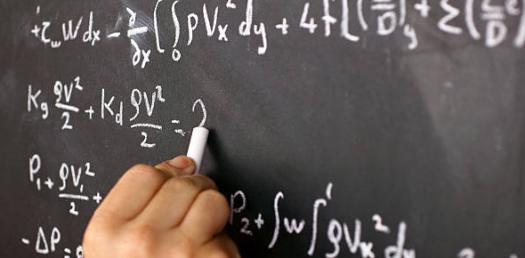# Math Skill Questions

8 Questions | Total Attempts: 55676SettingsSkills are something that people develop through a lot of practice and you can’t be a professional without the skills. The quiz below is designed to test your math skills and see if you are all that! Give it a try and see if you should polish up on some problems.

Related Topics
• 1.
Anosov automorphism is defined as a what?
• A.

Hyperbolic Linear Map

• B.

Integral

• C.

Derivative

• D.

Theory

• E.

Geometric Proof

• 2.
A box classified in geometric terms is a what?
• A.

A polyhedron.

• B.

A shape.

• C.

A polyhedron whose faces are all rectangles.

• D.

A polyhedron with multiple sides.

• E.

A rectangular prism.

• 3.
Elliptic sines are defined as what? Hint: It is represented as: S (a , 0 , z ) = sin (√a z )
• A.

Elliptic cosine defined as an odd Mathieu function ser(z , q ) with characteristic value ar.

• B.

Elliptic sine defined as an even Mathieu function ser(z , q' ) with characteristic value ar.

• C.

Euclydian geometry.

• D.

Elliptic sine defined as an odd Mathieu function ser(z , q ) with characteristic value ar.

• E.

None of the above.

• 4.
If a=b, b=c, c=d, d=?
• A.

D-(abc)

• B.

A

• C.

C+b

• D.

D-c

• E.

None of the above.

• 5.
Calculus can be applied as polynomials.
• A.

True

• B.

False

• C.

Can't be determined with the information give.

• 6.
If f(x) = x2, then...
• A.

F'(x) = x/2x

• B.

X = f'

• C.

F'(x) = 2x

• D.

F'(x) =2x(f'(x))

• E.

X = x(x=1)

• 7.
• A.

Let X be a normed space, M and N be algebraically complemented subspaces of X i.e. M+N = X and M ∩ N = {0}, Φ:X → X/M be the quotient map, Φ:MxN → X be the natural isomorphism (x,y)| → x+y, and P:X → M,P(x+y) = x,x ∈ M,y ∈ N

• B.

M ∩ N = {0}, Φ:X → X/M be the quotient map, Φ:MxN → X be the natural isomorphism (x,y)| → x+y, and P:X → M,P(x+y

• C.

X be the disk algebra, i.e., the space of all analytic functions on {z ∈ C:|z| < 1} which are continuous on the closure of D. Then the subspace of C(T) consisting of the restrictions of functions of X to T = {z ∈ C:|z| = 1} is not complemented in X.

• D.

(x,y)| → x+y, and P:X → M,P(x+y) = x,x ∈ M,y ∈ N be the projection of X on M along N.

• E.

None of the above?

• 8.
The following is an example of what?
• A.

Binary Bracketing

• B.

Abel's Binomial Theorem

• C.

Pascal's Formula

• D.

Stanley's Identity

• E.

Bernoulle Triangle